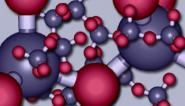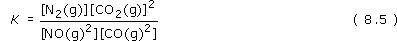Science, Maths & Technology

### Become an OU studentThe molecular world

Start this free course now. Just create an account and sign in. Enrol and complete the course for a free statement of participation or digital badge if available.

# 7.2.1 The equilibrium constant

An expression for the equilibrium constant of a reaction can be put together from the concentrations of the reactants and products at equilibrium. A concentration of a reactant or product is represented by enclosing its chemical formula in square brackets. Thus, the concentration of NO(g) is written [NO(g)].

To write down the equilibrium constant of a reaction, we start with the concentrations of the products. Each one is raised to the power of the number that precedes it in the reaction equation, and the corresponding terms for each product are then multiplied together.

## Question 44

Do this now for the products of the equilibrium system 8.2.

The result is [N2(g)] × [CO2(g)]2, or, taking the multiplication sign as understood, [N2(g)][CO2(g)]2. In Equation 8.2, CO2(g) is preceded by a two, so its concentration is squared.

## Question 45

Now repeat the operation for the reactants in Equation 8.2.

The result is [NO(g)]2[CO(g)]2; in Equation 8.2, both NO(g) and CO(g) are preceded by a two.

The equilibrium constant, K, is obtained by dividing the result for the products by the result for the reactants:We have raised the possibility that Reaction 8.1 does not happen because the equilibrium position for equilibrium system 8.2 lies well over to the left. In other words, at equilibrium, the concentrations of NO(g) and CO(g) are very high, and those of N2 (g) and CO2(g) are so small as to be undetectable.

## Question 46

If so, will K be large or small?